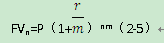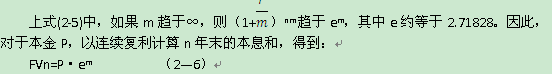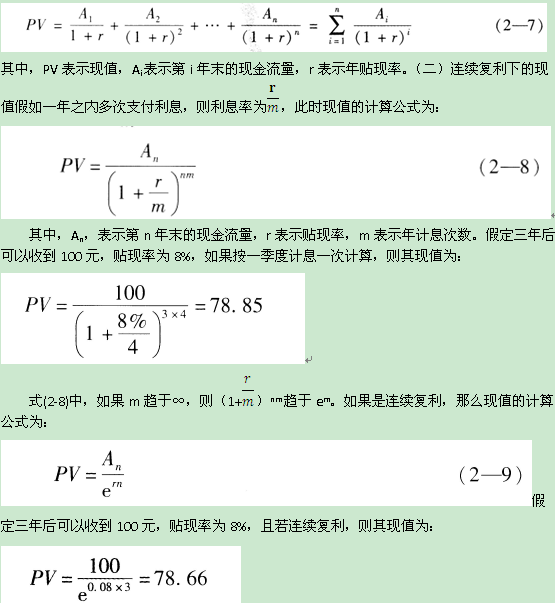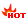# 2019年中级经济师金融专业核心考点:利率的计算

(一)单利

10000×0.5%×10=500(元)

(二)复利复利也称利滚刑，是将每一期产生的利息加入本金一并计算下一期的利息。复利的计算公式为：FV=P(1+r)n(2-3)I=FV-P=P[(1+r)n-1] (2-4)

FV1/2=100×(1+0.06÷2)=103(元)

FV1=100×(1+0.06÷2)2=106.09(元)

106.09元与一年计一次息的本息和106元比较，多出0.09元，这是因为对第一个6个月的利息3元计算利息的缘故，如果一年中计息次数增加，到年底本息和会越来越大。在上例中，如果一个季度支付一次利息，一年后的本息和为：FV1=100×(1+0.06÷4)4=106.14(元)如果一个月支付一次利息，一年后的本息和为：

FV1=100×(1+0.06÷12)12=106.17(元)一般来说，若本金为P，年利率为r，每年的计息次数为，n，则第n年末的本息和为：(三)连续复利(一)系列现金流的现值每年的计息次数越多，现值越小。随着计息间隔的缩短，现值以递减的速度减小，最后等于连续复利条件下的现值。

(三)终值及其计算

FVn=P+P·r·n=P(1+r·n) (2—10)

10000×(1+4%)10=14802.44(元)

>>经济师考试零基础一次过全科，槐俊升老师为你解密90%原题考点>>